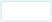﻿ EnergyCalculator2014_1
Energy Calculator (Conventional vs LED)
Instructions: Fill in all the grey boxes
100-175 Watt equivalent (BL-12RFP), choose 175   150-250 Watt equivalent (HC-11), choose 250
250-400 Watt equivalent (HC-22), choose 350   400-500 Watt equivalent (HC-22-3), choose 400
750 Watt equivalent (HC-33), choose 750   1000 Watt equivalent (HC-44), choose 10
Wattage of bulb
Number Of fixtures
Hours lights are on daily       =
Kilo Watt Hours   365 Days used   =
kW/h annually    kWh rate   =
Annual cost of operation (Conventional)
NCC HC-Series
Wattage of bulb  Number Of fixtures =
Hours lights are on daily       =
Kilo Watt Hours   365 Days used   =
kW/h annually    kWh rate   =
Annual cost of operation (HC-Series)
Annual energy savings